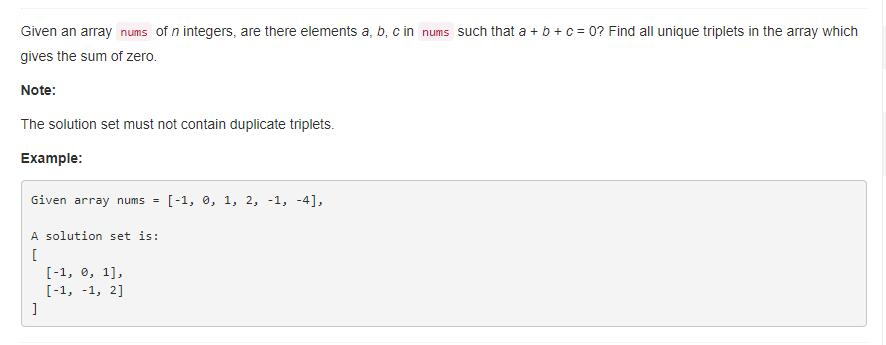# 题目描述（中等难度）# 解法一 暴力解法

public List<List<Integer>> threeSum(int[] nums) {
List<List<Integer>> res = new ArrayList<List<Integer>>();
for (int i = 0; i < nums.length; i++) {
for (int j = i + 1; j < nums.length; j++)
for (int k = j + 1; k < nums.length; k++) {
if (nums[i] + nums[j] + nums[k] == 0) {
List<Integer> temp = new ArrayList<Integer>();
//判断结果中是否已经有 temp 。
if (isInList(res, temp)) {
continue;
}
}
}
}
return res;

}

public boolean isInList(List<List<Integer>> l, List<Integer> a) {
for (int i = 0; i < l.size(); i++) {
//判断两个 List 是否相同
if (isSame(l.get(i), a)) {
return true;
}
}
return false;
}

public boolean isSame(List<Integer> a, List<Integer> b) {
int count = 0;
Collections.sort(a);
Collections.sort(b);
//排序后判断每个元素是否对应相等
for (int i = 0; i < a.size(); i++) {
if (a.get(i) != b.get(i)) {
return false;
}
}
return true;
}


# 解法二

public List<List<Integer>> threeSum(int[] num) {
Arrays.sort(num); //排序
for (int i = 0; i < num.length-2; i++) {
//为了保证不加入重复的 list,因为是有序的，所以如果和前一个元素相同，只需要继续后移就可以
if (i == 0 || (i > 0 && num[i] != num[i-1])) {
//两个指针,并且头指针从i + 1开始，防止加入重复的元素
int lo = i+1, hi = num.length-1, sum = 0 - num[i];
while (lo < hi) {
if (num[lo] + num[hi] == sum) {
//元素相同要后移，防止加入重复的 list
while (lo < hi && num[lo] == num[lo+1]) lo++;
while (lo < hi && num[hi] == num[hi-1]) hi--;
lo++; hi--;
} else if (num[lo] + num[hi] < sum) lo++;
else hi--;
}
}
}
return res;
}